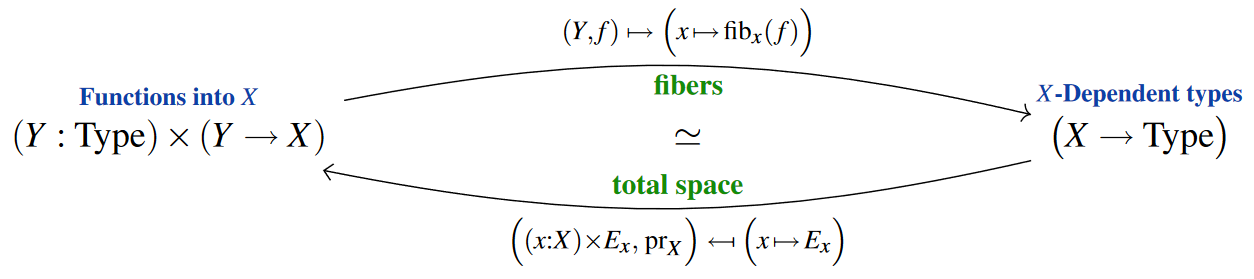Contents

# Contents

## Idea

In type theory, a dependent type or type in context is a family or bundle of types which vary over the elements (terms) of some other type. It can be regarded as a formalization of the notion of “indexed family,” providing a structural account of families (in contrast to the material approach which requires sets to be able to contain other sets as elements).

Type theory with the notion of dependent types is called dependent type theory.

In the categorical semantics of type theory, a dependent type

$x:A \; \vdash \;B(x) \;\mathrm{type}$

is represented by a particular morphism $p\colon B\to A$, the intended meaning being that each type $B(x)$ is the fiber of $p$ over $x\in A$. The morphism in a category that may represent dependent types in this way are sometimes called display morphisms (especially when not every morphism is a display morphism).

Dependent types can be thought of as fibrations (Serre fibrations) in classical homotopy theory. The base space is $X$, the total space is $\sum_{(x:X)}P(x)$ and the fiber $P(\star_X)$. This gives the fibration:

$P(\star_X)\to \sum_{x:X}P(x) \to X$

## Relation to families of elements.

One may understand $A$-dependent types $a \colon A \;\vdash\; B(a)$ equivalently as functions $B \to A$ with codomain $A$, i.e. as “type-bundles” (in the general, not in locally trivial sense of fiber bundles, though) or “type-fibrations” (in a rather accurate sense):

The domain $B$ — i.e. the “total space” of these bundles — is identified with the dependent sum of the “fibers$B(a)$, and conversely these fibers are indeed recovered from any such bundle as the fiber types.

Under the categorical semantics of dependent type theory (cf. categorical model of dependent types and relation between type theory and category theory) it is exactly these associated “type bundles” which are identified with actual fibrations (“display maps”) in the given semantic category.

We now say this in more detail, first

and then as

### Syntactically

Given a family of types, $x:A \vdash B(x)$, one can construct a family of elements $z:\sum_{x:A} B(x) \vdash \pi_1(z):A$ via the elimination rules for negative dependent sum types:

$\frac{\Gamma \vdash A \; \mathrm{type} \quad \Gamma, x:A \vdash B(x) \; \mathrm{type}}{\Gamma, z:\sum_{x:A} B(x) \vdash \pi_1(z):A}$

Conversely, given a family of elements $x:A \vdash f(x):B$ one can construct a family of types $y:B \vdash \sum_{x:A} f(x) =_B y$ as the family of fiber types of $x:A \vdash f(x):B$, via the formation rules for identity types and dependent sum types:

$\frac{\Gamma \vdash A \; \mathrm{type} \quad \frac{\Gamma \vdash A \; \mathrm{type} \quad \Gamma \vdash B \; \mathrm{type} \quad \Gamma, x:A \vdash f(x):B}{\Gamma, x:A, y:B \vdash f(x) =_B y \; \mathrm{type}}}{\Gamma, y:B \vdash \sum_{x:A} f(x) =_B y \; \mathrm{type}}$

This corresponds to the relation between type theory and category theory: there are two ways to interpret a morphism $f:\mathrm{Hom}_C(A, B)$ in a category $C$

• as a family of elements $x:A \vdash f(x):B$
• as a family of types $y:B \vdash A(y)$

If the type theory has function types, then the above notions are also interderivable with an element of a function type $f:A \to B$, which correspond to the internal hom of a category $C$.

One can additionally derive the following equivalences of types:

$p \;\;\;\; \colon \;\;\;\; A \;\; \simeq \; \sum_{y:B} \sum_{x:A} \big( f(x) =_B y \big)$
$y \colon B \;\;\;\; \vdash \;\;\;\; q(y) \;\;\; \colon \;\;\; A(y) \;\simeq\; \sum_{ \mathclap{ z \colon \sum_{x:B} A(x) } } \big( \pi_1(z) =_A y \big)$

### Reflected in the type universe

Assuming the univalence-axiom, the analogous statement holds reflected within a type universe $Type$: Given $X \,\colon\, Type$, the type $X \to Type$ of $X$-dependent types is equivalent to the type $(X \colon Type) \times (Y \to X)$ of functions with codomain $X$, via forming fiber types and dependent sum-types, respectively [UFP13, Thm. 4.8.6]

$X \colon Type \;\;\;\; \vdash \;\;\;\; (Y \colon Type) \times (Y \to X) \;\; \simeq \;\; \big( X \to Type \big) \,.$Under the categorical semantics of homotopy type theory in $\infty$-toposes, this characterizes the type universe as (interpreted by) the (small) object classifier in an $\infty$-topos.

## Examples

When the theory of a category is phrased in dependent type theory then there is one type “$obj$” of objects and a type $hom$ of morphisms, which is dependent on two terms of type $obj$, so that for any $x,y:obj$ there is a type $hom(x,y)$ of arrows from $x$ to $y$. This dependency is usually written as $x,y:obj \vdash hom(x,y):Type$. In some theories, it makes sense to say that the type of “$hom$” itself is $obj, obj\to Type$ (usually understood as $obj \to (obj \to Type)$ or $(\obj \times \obj) \to Type$), i.e. a function from pairs of elements of $A$ to the universe $Type$ of types.

See the references at dependent type theory.

Exposition:

In Coq:

• Yves Bertot?, Introduction to dependent types in Coq [pdf]

The relation between dependent types and bundles (functions of given codomain)